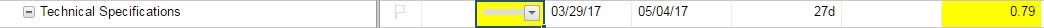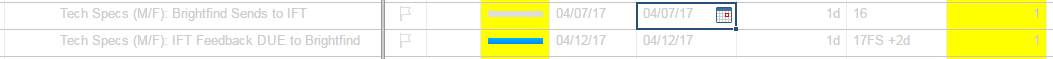#### Welcome to the Smartsheet Forum Archives

The posts in this forum are no longer monitored for accuracy and their content may no longer be current. If there's a discussion here that interests you and you'd like to find (or create) a more current version, please Visit the Current Forums.

# Progress Bar Automation, based on % Complete IF/THEN

I conducted a search but could not find this specific topic, so please forgive if I am repeating an existing question. Hopefully the subject line is incrementally more search optimized.

BACKGROUND

I am using IF/THEN formulas in my '% Complete' column to auto-populate a Progress Bar that goes into my status reports as a visual element.

The formulas that I have attempted are as follows:

1. =IF([% Complete]31 = 1, "Full", IF([% Complete]31 < 0.25, "Empty", IF([% Complete]31 = 0.2 - 0.49, "Quarter", IF([% Complete]31 = 0.5 - 0.69, "Half", IF([% Complete]31 = 0.7 - 0.99, "Three Quarter")))))
2. =IF([% Complete]1 = 1, "Full", IF([% Complete]1 < 0.25, "Empty", IF([% Complete]1 >= 0.75, "Three Quarter", IF([% Complete]1 >= 0.5, "Half", IF([% Complete]1 >= 0.25, "Quarter")))))

The formula below is what I am currently using. It is the same as #2 above, just in reverse order.

=IF([% Complete]1 = 1, "Full", IF([% Complete]1 < 0.25, "Empty", IF([% Complete]1 >= 0.25, "Quarter", IF([% Complete]1 >= 0.5, "Half", IF([% Complete]1 >= 0.75, "Three Quarter")))))

PROBLEM

Inconsistent value returns based on the same formula in different rows (see Capture2.png)

The correct value in % Complete does not appear to register in the formula (see Capture.png)

SEEKING

A formula solution that auto-populates the progress bar based on the % Complete system row.

< 25% is Empty

26% - 49% is Quarter

50% - 74% is Half

75% - 99% is Three Quarter

100% is Full

Any help or advice would be appreciated. Thanks!

EvanTags:

• One issue I can see in the formula that you are currently using is that your third IF statement will fire every time. A good thing to remember is that your IF statements will fire in order, and as soon as one of the IF statements are true, it will always end with that condition. In your case you, you should order your IF statements from FULL down to EMPTY. That way if you have data say, .35, it will fail your FULL, Three Quarter, and Half statements and then fire on your Quarter one.

Does that make sense? Let me know if you need help developing the query. The issue just appears to be in the order you are placing the IF statements.

• =IF(AND([% Complete]2 > 0, [% Complete]2 < 0.25), "Empty", IF(AND([% Complete]2 > 0.24, [% Complete]2 < 0.5), "Quarter", IF(AND([% Complete]2 > 0.49, [% Complete]2 < 0.75), "Half", IF(AND([% Complete]2 > 0.74, [% Complete]2 < 1), "Three Quarter", IF([% Complete]2 = 1, "Full")))))

• The AND's are redundant with a nested IF

=IF([% Complete]15 < 0.25, "Empty", IF([% Complete]15 < 0.5, "Quarter", IF([% Complete]15 < 0.75, "Half", IF([% Complete]15 < 1, "Three Quarter", IF([% Complete]15 = 1, "Full", "Unknown")))))

If you are worried about negative percentages, then an AND at the beginning would be OK.

Craig

This discussion has been closed.